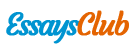# Independent and Dependent Variable

Autor:   •  June 27, 2017  •  Study Guide  •  712 Words (3 Pages)  •  300 Views

Page 1 of 3

Independent and dependent variable

==========

﻿Name: Gabriel Garcia de Carvalho 09/07/2014

PH 383

Biostatistics

Assignment 1

Due Date: September 9, 2014

1. Provide an explanation/definition of [6 pts]

a. Independent Variable:

The independent variable is the variable that is able to lead to changes in the status of the dependent variable. In fact, it is an event or condition that produce an effect. An independent variable can be manipulated by the researcher. In biostatistics, we must not call independent variable as a cause.

b. Dependent Variable:

The dependent variable is the presumed effect or response that is measured in an experiment or research. The dependent variable is not manipulated by the researcher, it is only a result of the variation in the independent variable.

2. What is/are the defining characteristic(s) of [4 pts]

1. Nominal Variable:

- Used primarily for categorizing data in two or more categories;

- frequently referred as qualitative;

- do not have a presumed order or numerical value associated, unless in statistical analysis;

- example: race, gender, color, etc.

b. Ordinal Variable:

- Represent an ordered series of relationship;

- applied as the rank orders or standings;

- qualitative variable;

- example: level of satisfaction, pain level, causes of death, etc.

c. Interval Variable:

- It can be measured along a continuum;

- have a numerical value;

- quantitative variable;

- can be in categories;

- we always have answers, we cannot have absence;

- we cannot compute ratios for interval variable;

- example: Fahrenheit scale.

d. Ratio Variable:

- Ratio variables are interval

...